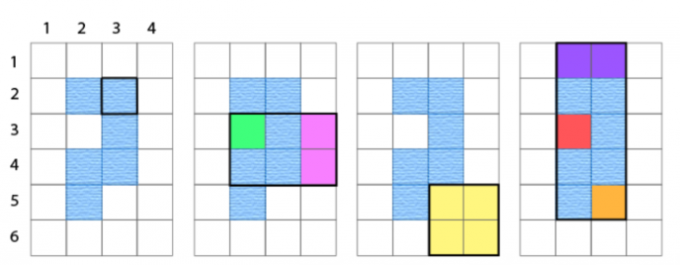# #303. 【APIO2017】斑斓之地

### 实现细节

• init(R, C, sr, sc, M, S) --- 该函数会在开始时调用并且只会调用一次

• R 和 C: 表示网格的行数和列数
• sr 和 sc: 彩虹蛇的起点坐标
• M: 彩虹蛇移动的步数
• S: 一个长度为 M 的字符串, S[i] 是N、S、E、W之一, 分别表示彩虹蛇的第 $i$ 次移动是向正北、正南、正东、正西移动一格，$0 \leq i\leq M - 1$ 。保证彩虹蛇不会移动到网格外面。
• colours(ar, ac, br, bc) --- 在调用init一次之后, 该函数会被总共调用 $Q$ 次

• $(a_r, a_c)$ 表示你所购买矩形的西北角的格子的坐标，$(b_r, b_c)$ 表示你所购买矩形的东南角的格子的坐标，返回一个整数表示在这种购买方案下最多能够将土地染上多少种不同的颜色
• $1 \leq a_r \leq b_r \leq R$, $1 \leq a_c \leq b_c \leq C$

### 样例测试程序

• 第1行:四个整数 $R$, $C$, $M$ 和 $Q$;
• 第2行:两个整数 $s_r$ 和 $s_c$;
• 第3行:一个包含 $M$ 个字符的字符串 $S$, 每个字符是N、S、E、W之一 (如果 $M = 0$ 则此行应留空);
• 第4行到 $Q+3$ 行:每行四个整数$a_r$, $a_c$, $b_r$ 和 $b_c$。

### 样例和解释

init(6, 4, 3, 3, 9, "NWESSWEWS")提供了网格的大小，彩虹蛇的起点和它的移动路径。
colours(2, 3, 2, 3)0只有 $(2, 3)$ 被矩形包含,但 $(2, 3)$ 是河，所以没有格子需要染色，颜色数为 $0$ 。
colours(3, 2, 4, 4)2河将矩形区域分成了两部分:一部分包含 $(3, 2)$，另一部分包含 $(3,4)$ 和 $(4,4)$ ;因此最多染 $2$ 种不同的颜色。
colours(5, 3, 6, 4)1矩形区域内不包含河，所有格点都是联通的，所以你最多染 $1$ 种 颜色。
colours(1, 2, 5, 3)3河将矩形区域分成了三部分:第一部分包含 $(1, 2)$ 和 $(1, 3)$ ，第二 部分包含 $(3, 2)$，第三部分包含 $(5, 3)$，因此最多可以染$3$种不同的颜色。### 样例输入

6 4 9 4
3 3
NWESSWEWS
2 3 2 3
3 2 4 4
5 3 6 4
1 2 5 3


### 样例输出

0
2
1
3


### 子任务

111 $R \leq 50$ $C \leq 50$ $Q \leq 1000$
212 $R = 2$ $C \leq 200000$ $Q \leq 100000$
324 $R = 200000$ $C \leq 200000$ $Q = 1$
427 $R = 1000$ $C \leq 1000$ $Q = 100000$
526 $R = 200000$ $C \leq 200000$ $Q = 100000$